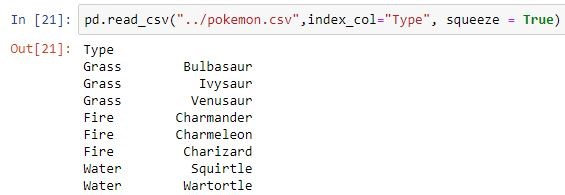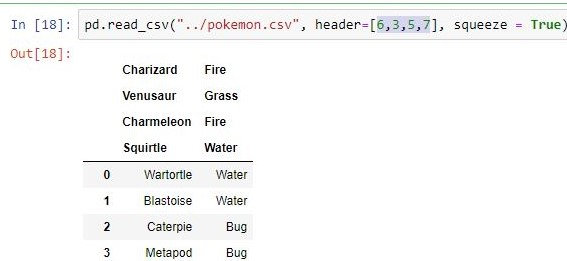Python is a great language for doing data analysis, primarily because of the fantastic ecosystem of data-centric python packages. Pandas is one of those packages and makes importing and analyzing data much easier.

Import Pandas:

`import pandas as pd`

Code #1 : read_csv is an important pandas function to read csv files and do operations on it.

 `# Import pandas ` `import` `pandas as pd ` ` `  `# reading csv file  ` `pd.read_csv(``"filename.csv"``) `

Opening a CSV file through this is easy. But there are many others thing one can do through this function only to change the returned object completely. For instance, one can read a csv file not only locally, but from a URL through read_csv or one can choose what columns needed to export so that we don’t have to edit the array later.

Here is the list of parameters it takes with their Default values.

pd.read_csv(filepath_or_buffer, sep=’, ‘, delimiter=None, header=’infer’, names=None, index_col=None, usecols=None, squeeze=False, prefix=None, mangle_dupe_cols=True, dtype=None, engine=None, converters=None, true_values=None, false_values=None, skipinitialspace=False, skiprows=None, nrows=None, na_values=None, keep_default_na=True, na_filter=True, verbose=False, skip_blank_lines=True, parse_dates=False, infer_datetime_format=False, keep_date_col=False, date_parser=None, dayfirst=False, iterator=False, chunksize=None, compression=’infer’, thousands=None, decimal=b’.’, lineterminator=None, quotechar='”‘, quoting=0, escapechar=None, comment=None, encoding=None, dialect=None, tupleize_cols=None, error_bad_lines=True, warn_bad_lines=True, skipfooter=0, doublequote=True, delim_whitespace=False, low_memory=True, memory_map=False, float_precision=None)

Not all of them are much important but remembering these actually save time of performing same functions on own. One can see parameters of any function by pressing shift + tab in jupyter notebook. Useful ones are given below with their usage :

Parameter Use
filepath_or_buffer URL or Dir location of file
sep Stands for seperator, default is ‘, ‘ as in csv(comma seperated values)
index_col Makes passed column as index instead of 0, 1, 2, 3…ruse_cols Only uses the passed col[string list] to make data frame
squeeze If true and only one column is passed, returns pandas series
skiprows Skips passed rows in new data frame

Refer the link to data set used from here.

Code #2 :

 `# importing Pandas library ` `import` `pandas as pd ` ` `  `pd.read_csv(filepath_or_buffer ``=` `"pokemon.csv"``) ` ` `  `# makes the passed rows header ` `pd.read_csv(``"pokemon.csv"``, header ``=``[``1``, ``2``]) ` ` `  `# make the passed column as index instead of 0, 1, 2, 3.... ` `pd.read_csv(``"pokemon.csv"``, index_col ``=``'Type'``) ` ` `  `# uses passed cols only for data frame ` `pd.read_csv(``"pokemon.csv"``, usecols ``=``[``"Type"``]) ` ` `  `# reutruns pandas series if there is only one colunmn ` `pd.read_csv(``"pokemon.csv"``, usecols ``=``[``"Type"``], ` `                              ``squeeze ``=` `True``) ` `                               `  `# skips the passed rows in new series ` `pd.read_csv(``"pokemon.csv"``, ` `            ``skiprows ``=` `[``1``, ``2``, ``3``, ``4``]) `

My Personal Notes arrow_drop_upDeveloper in day, Designer at night GSoC 2019 with Python Software Foundation (EOS Design system)

If you like GeeksforGeeks and would like to contribute, you can also write an article using contribute.geeksforgeeks.org or mail your article to contribute@geeksforgeeks.org. See your article appearing on the GeeksforGeeks main page and help other Geeks.

Please Improve this article if you find anything incorrect by clicking on the "Improve Article" button below.

Article Tags :

8

Please write to us at contribute@geeksforgeeks.org to report any issue with the above content.